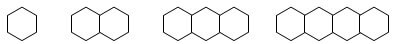# Mathematics Grade 4 Practice Questions

##### 1. Which statement is false?
1. 7 pens of 3 cattle is 20 cattle.
2. 8 pens of 3 cattle is 24 cattle.
3. 3 containers filled with 8 pencils is 24 pencils.
4. 3 containers filled with 7 pencils is 21 pencils.
1. \$0.20
2. \$0.25
3. \$0.30
4. \$0.35
1. 0
2. 1
3. 2
4. 3
1. 12
2. 24
3. 60
4. 80
##### 5. Which pattern of numbers describes the number of line segments needed in the shape pattern listed below?1. 1, 2, 3, 4
2. 6, 12, 18, 24
3. 6, 10, 14, 18
4. 6, 11, 16, 21
##### 6. Which of the following statements is true?
1. 800 is ten times greater than 8.
2. 3000 is ten times greater than 30.
3. 70 is ten times greater than 700.
4. 900 is ten times greater than 90.
##### 7. Which of the following is the written form for the number 5,320,080?
1. five million, thirty-two thousand, eighty
2. five million, three hundred twenty thousand, eighty
3. five million, three hundred twenty thousand, eight
4. five thousand, three hundred twenty-eight
1. 7,850
2. 7,860
3. 7,800
4. 7,900
1. 740
2. 840
3. 830
4. 930
##### 10. Evaluate 1250-487.
1. 837
2. 737
3. 763
4. 863

1. A: If there are 7 pens with 3 cattle, then the total number of cattle is found by 7×3=21, not 20. In the other problems, 8×3=24,3×8=24, and 3×7=21.

2. B: The cost of one eraser is found by dividing the total cost by the number of erasers. Since the cost of the erasers is \$4.50, and there are 18 erasers, the expression for the cost of the eraser will be \$4.50×18=\$0.25. The total cost of one eraser is \$0.25

3. C: If Raquel had 36 stamps and divided them into 9 groups, there will be 4 stamps per group because 36×9=4. She then adds 2 stamps to each group so that she has 6 stamps per group. Since there are nine groups of six and 9×6=54, she has a total of 54 stamps. If she gives 4 stamps each to 13 friends, she gives away 4×13=52 stamps. This means she has 54-52=2 stamps left over.= 13 r 2, so she gave four stamps to each of her 13 friends and had a remainder of 2

4. C: One number is the factor of another if it will divide it with no remainder. Ten is not a factor of twelve because 12×10=1 remainder 2. Also, ten is not a factor of twenty-four because 24×10=2 remainder 4. Six is not a factor of eighty because 80×6=13 remainder 2. The correct answer is sixty, because 4, 6 and 10 all divide it evenly.

5. D: The first figure had 6 sides. The second figure has 11 sides because 5 sides had to be added to make the next figure. This pattern of adding 5 segments continued, so the answers had to be 6, 11, 16, and 21.

6. D: 90×10=900, so 900 is 10 times greater than 90. 800 is 100 times greater than 8, 3000 is 100 times greater than 30, and 70 is only a tenth of 700. Therefore the only answer is D.

7. B: The value of A is 5,032,080; the value of C is 5,320,008; and the value of D is 5,328. B shows 5,320,080 which is the original number.

8. D: The 8 is in the hundreds place, and because the number to the right of 8 is a five, the 8 must round up to a 9. The remaining numbers change to a 0. Therefore the answer is 7,900.

9. B: Adding the ones place, you get 10, so the one carries to the tens place. 1+8+5=14, so the 4 goes in the tens place and the 1 is carried over the hundreds place. 1+4+3=8, so the 8 goes into the hundreds place. The final answer is 840. 452
+388
840

10. C: To subtract the ones column, a 1 has to be borrowed from the tens column, so the 0 becomes 10 and the 5 becomes a 4. 10-7=3, so the 3 goes in the ones unit. To subtract the tens column, a 1 needs to be borrowed from the 2 in the hundreds column. That 2 becomes 1 and the 4 in the tens column becomes 14. 14-8=6, so the 6 goes in the tens column. Finally the 1 is borrowed from the thousands column so that 1 becomes 0 in the thousands column and the 1 in the hundreds column becomes 11. 11-4=7 so the 7 goes in the hundreds place of the answer. The final answer is 763. 1250
-487
763